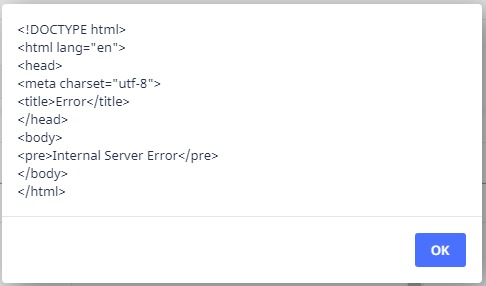1

# do as... massive speed improvement

Hi All

Has anyone seen the documentation about speed improvements in Ninox?

https://docs.ninox.com/en/use-cases/tips-and-tricks-for-fast-databases

I tested their use of do as...

This code took 2 mins 23 secs to run

``````for i in range(1000) do
create Test
end
``````

Whereas this code took less than 2 seconds to run!

``````do as transaction
for i in range(1000) do
create Test
end
end
``````

## 4replies• Fred
• 3 mths ago
• Reported - view

I thought the do as Transaction would be a great command, but sometime I get the following error on the web version of Ninox:Nothing that is show stopping, but annoying.

It is interesting that I find that the Ninox app on my new M2 MacBook Pro does things faster than the browser version. Tables and dashboards load faster. Even scripts run faster, that is why I wanted to use do as Transaction as that allows Ninox to use the app on my MBPro when I use it and on the server when I'm on my windows machine.

• Rafael Sanchis
• Rafael_Sanchis
• 3 mths ago
• Reported - view

Fred Hi Fred, How can I implement the do as server in this script. Work perfect on Android but too slow on Windows

``````let vDd := CutOff_;
let check := dialog(" Warning ", " ¿Confirm the Update Progress Project for Goole Graph ? ", ["Yes", "No"]);
if check = "Yes" then
for loop1 in select CutOff do
CutOff_ := loop1.DateCO;
let xPry := first(select Project);
let xFld1 := xPry.'Data|Date';
let xFld2 := xPry.Week;
let xFld3 := xPry.'Plan Value PV';
let xFld4 := xPry.'Earned Value EV';
let xFld5 := xPry.'Plan Hours';
let xFld6 := xPry.'Earned Hours';
let xFld7 := xPry.'% Plan';
let xFld8 := xPry.'% Earned';
let xFld9 := xPry.CPI;
let xFld10 := xPry.SPI;
let xFld11 := xPry.'Actual Cost AC';
let xFld12 := xPry.'Cost Week';
let xFld13 := xPry.'Cumulative Cost';
let xFld14 := xPry.'Actual Hours';
let xFld15 := xPry.'Hours Week';
let xFld16 := xPry.'Cumulative Hours';
let xFld17 := xPry.'Ideal Burdown';
let xFld18 := xPry.'Remaining Effort';
let Pgr := (create 'Progress-Cost Report');
Pgr.('Date CutOff' := xFld1);
Pgr.('Week CutOff' := xFld2);
Pgr.('Total Earned Plan' := xFld3);
Pgr.('Total Earned Real' := if cnt((select 'Progress-Cost Report')['Total Earned Real' = xFld4]) > 0 then
null
else
xFld4
end);
Pgr.(Plan_Hours := xFld5);
Pgr.(Earned_Hours := if cnt((select 'Progress-Cost Report')[Earned_Hours = xFld6]) > 0 then
null
else
xFld6
end);
Pgr.(Plan := xFld7);
Pgr.(Real := if cnt((select 'Progress-Cost Report')[Real = xFld8]) > 0 then
null
else
xFld8
end);
Pgr.(CPI := xFld9);
Pgr.(SPI := xFld10);
Pgr.('Actual Cost' := xFld11);
Pgr.('Cost Week' := xFld12);
Pgr.('Cumulative Cost' := if cnt((select 'Progress-Cost Report')['Cumulative Cost' = xFld13]) > 0 then
null
else
xFld13
end);
Pgr.('Actual Hours' := xFld14);
Pgr.('Hours Week' := xFld15);
Pgr.('Cumulative Hours' := if cnt((select 'Progress-Cost Report')['Cumulative Hours' = xFld16]) > 0 then
null
else
xFld16
end);
Pgr.(Burdown := xFld17);
Pgr.(Remaining := if cnt((select 'Progress-Cost Report')[Remaining = xFld18]) > 0 then
null
else
xFld18
end)
end
else
closeRecord()
end;
(select 'CutOff Date').(CutOff_ := vDd);
• Fred
• 3 mths ago
• Reported - view

You can try putting it after the if check = "Yes" line, remember to add an extra end at the bottom. Since you have dialog and alerts you can't wrap the whole thing in a do as server. I'm not sure if Ninox can pass things along between client and server properly, but you can try it out.

• Mel_Charles
• 3 mths ago
• Reported - view

Yep

Using do as ... does make a considerable difference - especially if you are on the web version and have big data tables, cos all the processing is done at the server end before handing it back for display etc.

## Content aside

• 1 Likes
• 3 mths agoLast active
• 4Replies
• 92Views
• 4 Following